Next: Example Eclipse Calculations Up: Lunar-Solar Syzygies and Eclipses Previous: Example Syzygy Calculations

# Solar and Lunar Eclipses

A solar eclipse--or, more accurately, a lunar-solar occultation--occurs when the moon blocks the light of the sun. Clearly, this is only possible at a new moon--see Fig. 26. On the other hand, a lunar eclipse occurs when the moon falls into the shadow of the earth. Of course, this is only possible at a full moon. It follows that eclipses can only take place at lunar-solar syzygies.

In order to determine whether a particular lunar-solar syzygy conincides with an eclipse, we first need to calculate the angular radii of the sun, the moon, and the earth's shadow in the sky. Using the small angle approximation, the angular radius of the sun is given by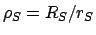, where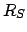is the solar radius, andthe earth-sun distance. However,, where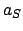,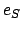, and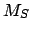are the major radius, eccentricity, and mean anomaly of the sun's apparent orbit around the earth, respectively (see Cha. 4). Hence,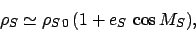(140)

where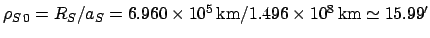. Likewise, the angular radius of the moon is(141)

where. Here,,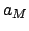,, andare the radius of the moon, and the major radius, eccentricity, and mean anomaly of the moon's orbit, respectively. As was shown in the previous chapter, lunar parallax causes the angular position of the moon in the sky to shift by up to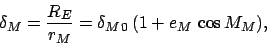(142)

where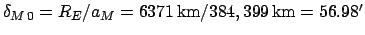. Here,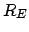is the radius of the earth. Finally, simple trigonometry reveals that the angular size of the earth's shadow (i.e., umbra) at the radius of the moon's orbit is(143)

This can be seen from Fig. 27. The radius of the umbra at the position of the moon is. Hence, the angular radius of the umbra is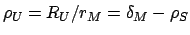. Incidentally, the identification of two of the angles in the figure withfollows because.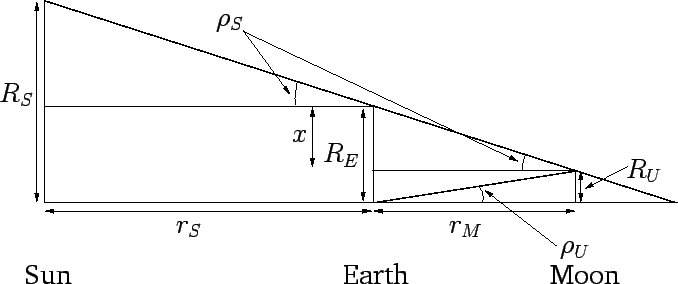A solar eclipse does not take place every new moon, nor a lunar eclipse every full moon, because of the inclination of the moon's orbit to the ecliptic plane, which causes the moon to pass either above or below the sun, or the earth's shadow, respectively, in the majority of cases. It follows that the critical parameter which determines the occurrence of eclipses is the ecliptic latitude of the moon at syzygy,. Of course, once the date and time of a syzygy has been established,can be calculated from Table 38. However, the lunar argument of latitude,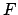, must first be determined using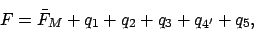(144)

wherecomes from Table 40,,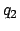,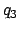, and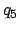are obtained from Table 41, andis thefrom Table 37. For instance, we have seen that for the third new moon of 1982 CE,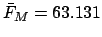,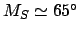,,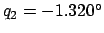,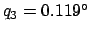, and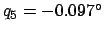. According to Table 37,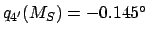. Hence,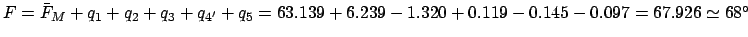. It follows from Table 38 that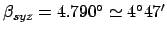.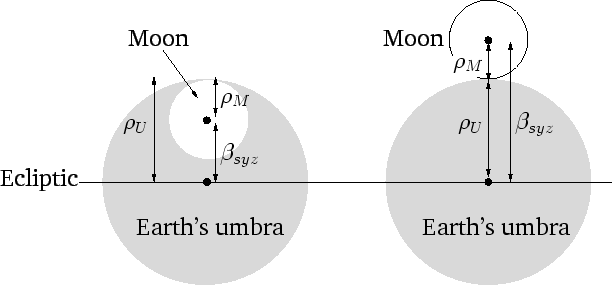The criterion for a lunar eclipse is particularly simple, since it is not complicated by lunar parallax. A total lunar eclipse, in which the moon is completely immersed in the earth's shadow, must take place at a full moon if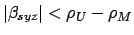(see Fig. 28), or equivalently(145)

and either a total or a partial lunar eclipse, in which the moon is only partially immersed in the earth's shadow, must take place if(see Fig. 28), or equivalently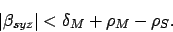(146)

Note that lunar eclipses are simultaneously visible at all observation sites on the earth for which the moon is above the horizon, since the earth's shadow is larger than the moon, and the relative position of the moon and the earth's shadow is not affected by parallax (since both the moon and the shadow are the same distance from the earth).

The criterion for a solar eclipse is modified by lunar parallax, which causes the angular position of the moon relative to the sun to shift by up tofrom its geocentric position. The amount of the shift depends on the observation site. However, a site can always be found at which the shift takes its maximum value in any particular direction. Note that the sun has negligible parallax, since it is much further from the earth than the moon. Taking parallactic shifts into account, a total solar eclipse, in which the sun is totally obscured by the moon, must take place ifand(147)

an annular solar eclipse, in which all of the sun apart from a thin outer ring is obscured by the moon, must take place if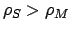and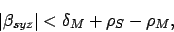(148)

and either a total, an annular, or a partial solar eclipse, in which the sun is only partially obscured by the moon, must take place if(149)

As a consequence of lunar parallax, and the fact that the angular sizes of the sun and moon in the sky are very similar, solar eclipses are only visible in very localized regions of the earth. Note, finally, that the above criteria represent necessary, but not sufficient, conditions for the occurrence of the various eclipses with which they are associated. This is the case because the point of closest approach of the moon and the earth's shadow, in the case of a lunar eclipse, and the moon and sun, in the case of a solar eclipse, does not necessarily occur exactly at the syzygy, due to the inclination of the moon's orbit to the ecliptic. However, since the said inclination is fairly gentle, the above criteria turn out to be very accurate predictors of eclipses.

The criterion for a total lunar eclipse can be written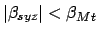, where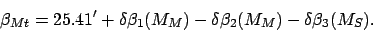(150)

Here, the functions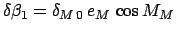,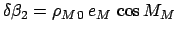, and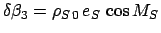are tabulated in Table 43. The criterion for any type of lunar eclipse becomes, where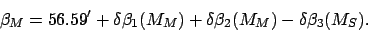(151)

The criterion for a total solar eclipse can be writtenand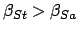, where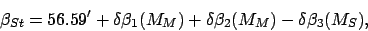(152)

and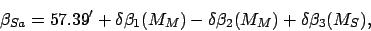(153)

The criterion for an annular solar eclipse isand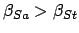. Finally, the criterion for any type of solar eclipse is, where(154)Next: Example Eclipse Calculations Up: Lunar-Solar Syzygies and Eclipses Previous: Example Syzygy Calculations
Richard Fitzpatrick 2010-07-21